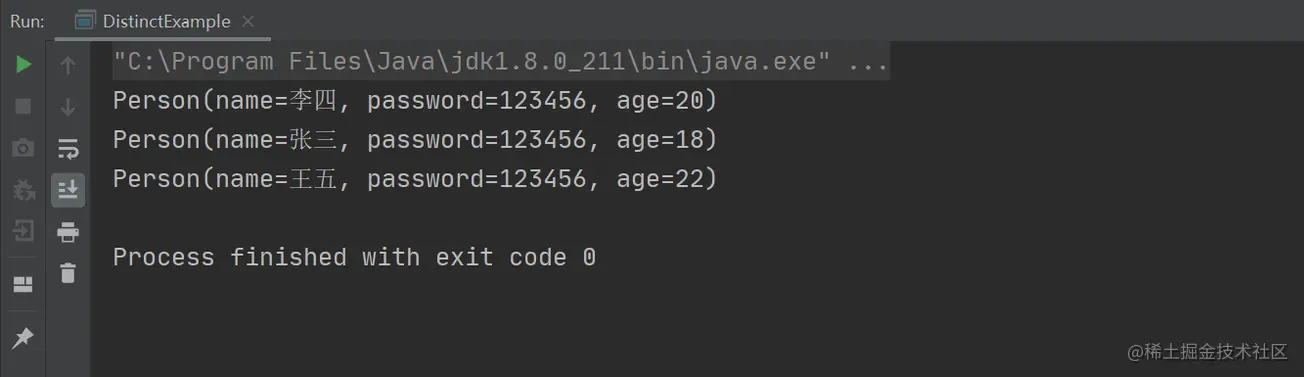# java 中如何實現 List 集合去重

List 去重指的是將 List 中的重復元素刪除掉的過程。此題目考察的是對 List 迭代器、Set 集合和 JDK 8 中新特性的理解與靈活運用的能力。

List 去重有以下 3 種實現思路：

• 自定義方法去重，通過循環判斷當前的元素是否存在多個，如果存在多個，則刪除此重復項，循環整個集合最終得到的就是一個沒有重復元素的 List；
• 使用 Set 集合去重，利用 Set 集合自身自帶去重功能的特性，實現 List 的去重；
• 使用 JDK 8 中 Stream 流的去重功能。

## 1.自定義去重

```import lombok.Data;
import java.util.ArrayList;
import java.util.List;
public class DistinctExample {
public static void main(String[] args) {
// 創建並給 List 賦值
List<Person> list = new ArrayList<>();
// 去重操作
List<Person> newList = new ArrayList<>(list.size());
list.forEach(i -> {
if (!newList.contains(i)) { // 如果新集合中不存在則插入
}
});
// 打印集合
newList.forEach(p -> System.out.println(p));
}
}
@Data
class Person {
private String name;
private int age;

public Person(String name, String password, int age) {
this.name = name;
this.age = age;
}
}``````import lombok.Data;
import java.util.ArrayList;
import java.util.Iterator;
import java.util.List;
public class DistinctExample {
public static void main(String[] args) {
// 創建並給 List 賦值
List<Person> list = new ArrayList<>();
// 去重操作
Iterator<Person> iterator = list.iterator();
while (iterator.hasNext()) {
// 獲取循環的值
Person item = iterator.next();
// 如果存在兩個相同的值
if (list.indexOf(item) != list.lastIndexOf(item)) {
// 移除相同的值
iterator.remove();
}
}
// 打印集合信息
list.forEach(p -> System.out.println(p));
}
}
@Data
class Person {
private String name;
private int age;

public Person(String name, String password, int age) {
this.name = name;
this.age = age;
}
}```## 2.利用 Set 集合去重

Set 集合天生具備去重特性，在創建 Set 集合時可以傳遞一個 List 集合，這樣就能實現數據轉移和去重的功能瞭，具體實現代碼如下：

```import lombok.Data;
import java.util.ArrayList;
import java.util.HashSet;
import java.util.List;
public class DistinctExample {
public static void main(String[] args) {
// 創建並給 List 賦值
List<Person> list = new ArrayList<>();
// 去重操作
HashSet<Person> set = new HashSet<>(list);
// 打印集合信息
set.forEach(p -> System.out.println(p));
}
}
@Data
class Person {
private String name;
private int age;

public Person(String name, String password, int age) {
this.name = name;
this.age = age;
}
}``````import lombok.Data;
import java.util.ArrayList;
import java.util.List;
public class DistinctExample {
public static void main(String[] args) {
// 創建並給 List 賦值
List<Person> list = new ArrayList<>();
// 去重操作
// 打印集合信息
set.forEach(p -> System.out.println(p));
}
}

@Data
class Person {
private String name;
private int age;

public Person(String name, String password, int age) {
this.name = name;
this.age = age;
}
}```## 3.使用 Stream 去重

```import lombok.Data;
import java.util.ArrayList;
import java.util.List;
import java.util.stream.Collectors;
public class DistinctExample {
public static void main(String[] args) {
// 創建並給 List 賦值
List<Person> list = new ArrayList<>();
// 去重操作
list = list.stream().distinct().collect(Collectors.toList());
// 打印集合信息
list.forEach(p -> System.out.println(p));
}
}
@Data
class Person {
private String name;
private int age;

public Person(String name, String password, int age) {
this.name = name;
this.age = age;
}
}```